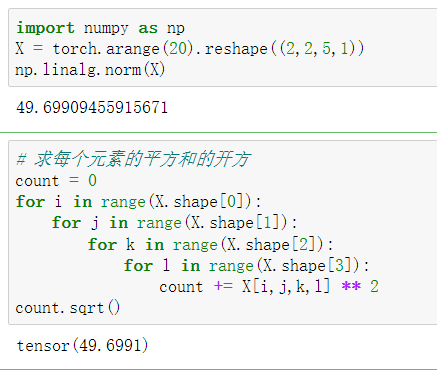# 线性代数

2022-05-15T16:00:00Z

C.sum(axis=2)很好理解。但是要计算C.sum(axis=0)时，可以写出C,C，用C+C。这是个人理解。但是碰到axis=1的时候，这种方法理解起来又出现了新的问题。比入到底应该 C+C+C;还是应该 C+C+C+ C+C+C。

tips：
1.还是建议在学习之前把整个知识点绘图为思维导图
2.对于学习是很容易忘记的，确定要进入该行业，对于知识点在大脑清晰的脉络还是非常importance
3.This is perfect deep learn’s book ，I have interesting in dl field

axis=0 ,可以理解为按行的方向进行压缩，那么就等价于按列求和，axis=1 理解为按列的方向进行压缩。不过如果维度过多axis=2，3这种，就不太好等价为按什么进行求和了

``````(4,3)
(3)
``````

Python里axis=0，是指运算沿着 ↓ 方向（向下）进行的；
axis=1，是指运算沿着 → 方向（向右）进行的。

sum(axis=1)的结果就是把每一行求和；

1，2，3：用线性代数证明。
4.答：输出是2。
5.答：是的。对应于形状中第一个轴的长度。
6.答：发生错误，因为形状不符合广播机制。广播机制是从形状尾部开始进行匹配，因此如果是A/A.sum(axis=1, keepdim=True)，此时两者形状为(5, 4)和(5, 1)，可以进行广播。
7.答：分别在轴0，1，2上降维，形状分别为(3, 4)、(2, 4)、(2, 3)。
8.答：均为一个标量。

0代表行的维度“消失了”，故只剩下一行，也就是列和；
1代表列的维度“消失了”，故只剩下一列，也就是行和；

``````In : A
Out:
tensor([[ 0.,  1.,  2.,  3.],
[ 4.,  5.,  6.,  7.],
[ 8.,  9., 10., 11.],
[12., 13., 14., 15.],
[16., 17., 18., 19.]])

In : B
Out:
tensor([[1., 1., 1.],
[1., 1., 1.],
[1., 1., 1.],
[1., 1., 1.]])

In : torch.mm(A, B)
Out:
tensor([[ 6.,  6.,  6.],
[22., 22., 22.],
[38., 38., 38.],
[54., 54., 54.],
[70., 70., 70.]])

In : A @ B
Out:
tensor([[ 6.,  6.,  6.],
[22., 22., 22.],
[38., 38., 38.],
[54., 54., 54.],
[70., 70., 70.]])
``````

1.使用数学方法证明A = (A’)’ 即可，比较简单，不重复赘述
2.同上，使用数学方法证明 (A + B)’ = A’ + B’即可
3.同上，对于n*n方阵中的任一下标i,j元素，都可证明 Aij + Aji = Aij + Aji = Bij = Bji。其中B = A + A’
4.len(X)输出的是2
5.与4相同，一起解释。假设X为四维张量，当张量X 的shape为 m * n * q * l 时，len(X)的长度为m，对应dim=0的长度，即shape的大小
6.运行 A/A.sum(axis=1) 会报错，报错原因如下：
假设A是shape为 m * n * q 的三维张量
则A.sum(axis=1)的 shape 为 m * q 的二维张量
我们知道，进行/运算时，要保证shape的大小是一致的，所以上式在运行时报错。
7. shape为(2,3,4)的形状，在dim=0时的输出shape为 (3,4),在dim=1时的输出shape为(2,4),在dim=2时的输出shape为(2,3)
8.提供一个四维的张量，经计算，输出的是一个标量，为L2范数，如下例子证明：